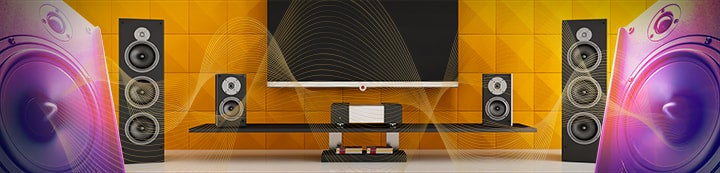1 - 6 of 6 Posts

#### apilon

·
##### Registered
Joined
·
266 Posts
Discussion Starter · ·
Good afternoon

Am i understanding correctly that if i want to set a butterworth 4th order in minidsp then i need to set the slope to 24db/oct and 48db/oct would be considered buttrworth 6th order?

Reason i am asking is i was reading about setting hpf for a vented sub and the article mentionned that you should set the filter to 1/2 octave so since my sub port is tune to 23hz 1/2 octave is 17.25hz but at 2nd order butterworth the cone excursion goes way pass the xmax of 29 mm so i need to set it at a 4th order butterworth to get it under xmax value

Alain

#### Wayne A. Pflughaupt

·
##### Super Moderator
Joined
·
9,309 Posts
If you are asking if 48 dB/octave is a 6th order slope, the answer is no – 6th order would be 36 dB/octave. 48 dB/octave is 8th order.

Regards,
Wayne

#### apilon

·
##### Registered
Joined
·
266 Posts
Discussion Starter · ·
If you are asking if 48 dB/octave is a 6th order slope, the answer is no – 6th order would be 36 dB/octave. 48 dB/octave is 8th order.

Regards,
Wayne
Thanks so what would be 4th order then ?

12db is 2nd order right ?

Alain

#### JohnM

·
Joined
·
6,711 Posts
Multiply the order by 6 to get the slope.

#### apilon

·
##### Registered
Joined
·
266 Posts
Discussion Starter · ·
Multiply the order by 6 to get the slope.
Thank for the explanation

so if in minidsp i set hpf to butterworth 48db/oct i just then set a 8th order butterworth if i understand correctly

#### AudiocRaver

·
##### Banned
Joined
·
4,838 Posts
Thank for the explanation

so if in minidsp i set hpf to butterworth 48db/oct i just then set a 8th order butterworth if i understand correctly
Correct.

1 - 6 of 6 Posts